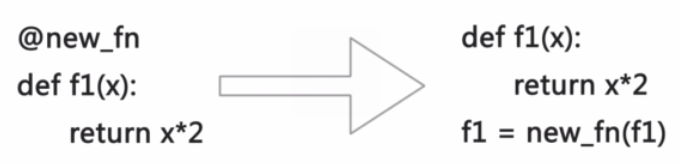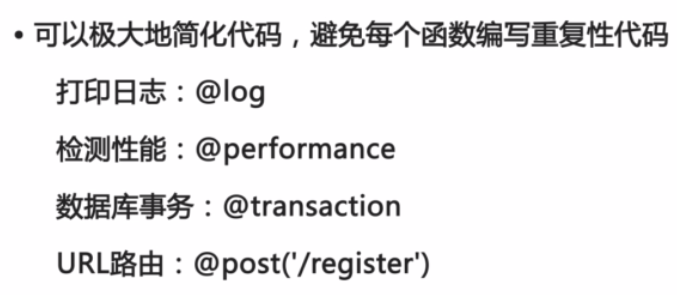# [python进阶课程] 函数式编程

[TOC]

http://www.imooc.com/learn/317

• 不需要变量 (python允许有变量, 所以python非纯函数式)
• 高阶函数
• 闭包: 返回函数
• 匿名函数

## 高阶函数

• 变量可以指向函数 `f=abs; f(-10)`
• 函数名: 就是指向函数的变量 `abs=len`
• 高阶函数: 接收函数作为参数的函数

def add(x,y,f):
return f(x)+f(y)
add(-5, 9, abs)

### map()

`map()`是 Python 内置的高阶函数，它接收一个函数 f 和一个 list，并通过把函数 f 依次作用在 list 的每个元素上，得到一个新的 list 并返回。map()函数不改变原有的 list，而是返回一个新的 list。

```def format_name(s):
return s.title()
print map(format_name, ['adam', 'LISA', 'barT'])
```

### reduce()

`reduce()`函数也是Python内置的一个高阶函数。reduce()函数接收的参数和 map()类似，一个函数 f，一个list，但行为和 map()不同，reduce()传入的函数 f 必须接收两个参数，reduce()对list的每个元素反复调用函数f，并返回最终结果值。

`reduce(function, iterable[, initializer])`
Apply function of two arguments cumulatively to the items of iterable, from left to right, so as to reduce the iterable to a single value. If the optional initializer is present, it is placed before the items of the iterable in the calculation, and serves as a default when the iterable is empty. If initializer is not given and iterable contains only one item, the first item is returned.

### filter()

filter()函数接收一个函数 f 和一个list，这个函数 f 的作用是对每个元素进行判断，返回 True或 False，filter()根据判断结果自动过滤掉不符合条件的元素，返回由符合条件元素组成的新list。

### 自定义sorted()

sorted()也是一个高阶函数，它可以接收一个比较函数`cmp`来实现自定义排序，比较函数的定义是，传入两个待比较的元素 x, y，如果 x 应该排在 y 的前面，返回 -1，如果 x 应该排在 y 的后面，返回 1。如果 x 和 y 相等，返回 0。

### 返回函数

```def calc_sum(lst):
def lazy_sum():
return sum(lst)
return lazy_sum
```

```>>> f = calc_sum([1, 2, 3, 4])
>>> f
<function lazy_sum at 0x1037bfaa0>
```

```>>> f()
10
```

### 闭包

```def calc_sum(lst):
def lazy_sum():
return sum(lst)
return lazy_sum
```

ex:

```# 希望一次返回3个函数，分别计算1x1,2x2,3x3:
def count():
fs = []
for i in range(1, 4):
def f():
return i*i
fs.append(f)
return fs
f1, f2, f3 = count()
```

```def count():
fs = []
for i in range(1, 4):
def f(j=i):
return j*j
fs.append(f)
return fs
f1, f2, f3 = count()
print f1(), f2(), f3()
```

### 匿名函数

Python中，对匿名函数提供了有限支持。

```map(lambda x: x * x, [1, 2, 3, 4, 5, 6, 7, 8, 9])
myabs = lambda x: -x if x < 0 else x
>>> myabs(-1)
1
```

## 装饰器

ex. 希望函数调用时打印调用日志⇒ 方法: 高阶函数: 接收要修改的函数, 进行包装后返回包装过的新函数.

```def new_f(f):
def fn(x):
print 'call %s()' % f.__name__
return f(x)
return fn
```(注意: 右边代码, 原本未装饰的f1函数已经被彻底隐藏了. )### 无参数decorator

def log(f):
def fn(*args, kw):
print 'call %s() in %s'%( f.name, time.ctime() )
return f(*args,

kw)
return fn

### 带参数decorator

```@log('DEBUG')
def my_func():
pass
```

`my_func = log('DEBUG')(my_func)`

```log_decorator = log('DEBUG')
my_func = log_decorator(my_func)
```

```log_decorator = log('DEBUG')
@log_decorator
def my_func():
pass
```

```def log(prefix):
def log_decorator(f):
def wrapper(*args, **kw):
print '[%s] %s()...' % (prefix, f.__name__)
return f(*args, **kw)
return wrapper
return log_decorator
@log('DEBUG')
def test():
pass
print test()
```

### 完善decorator

• 在没有decorator的情况下，打印函数名：
```def f1(x):
pass
print f1.__name__
```

⇒ 输出： f1

• 有decorator的情况下，再打印函数名：
```def log(f):
def wrapper(*args, **kw):
print 'call...'
return f(*args, **kw)
return wrapper
@log
def f2(x):
pass
print f2.__name__
```

⇒ 输出： wrapper

```def log(f):
def wrapper(*args, **kw):
print 'call...'
return f(*args, **kw)
wrapper.__name__ = f.__name__
wrapper.__doc__ = f.__doc__
return wrapper
```

```import functools
def log(f):
@functools.wraps(f)
def wrapper(*args, **kw):
print 'call...'
return f(*args, **kw)
return wrapper
```

`functools.wraps(f)`是一个装饰器函数, 目的是为了把最后返回的函数再次装饰(复制f的属性进去)... 所以对于带参数的装饰器, 应该在最里面返回的wrapper函数前加上`@functools.wraps(f)`

```import time, functools
def performance(unit):
def perf_decorator(f):
@functools.wraps(f)
def wrapper(*args, **kw):
print 'call %s() in %s %s'%( f.__name__, time.time(), unit ) #closure
return f(*args, **kw)
return wrapper
return perf_decorator
@performance('ms')
def factorial(n):
return reduce(lambda x,y: x*y, range(1, n+1))
print factorial.__name__
```

### 偏函数

```def int2(x, base=2):
return int(x, base)
```

`functools.partial`可以把一个参数多的函数变成一个参数少的新函数，少的参数需要在创建时指定默认值，这样，新函数调用的难度就降低了。

`functools.partial(func[,*args][, **keywords])`
Return a new partial object which when called will behave like func called with the positional arguments args and keyword arguments keywords.

```import functools
int2 = functools.partial(int, base=2)
>>> int2('1000000')
64
>>> int2('1010101')
85

sorted_ignore_case = functools.partial(sorted, key=lambda s:s.lower())
print sorted_ignore_case(['bob', 'about', 'Zoo', 'Credit'])
```

#### Part 1 of series «python进阶课程»：

comments powered by Disqus